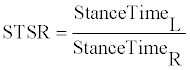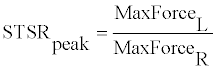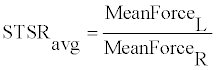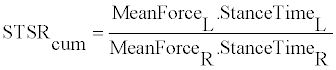Prediction of Stance Time and Force Symmetries using Instrumented Shoe Insoles for Use in Rehabilitation and Weight-Bearing Regimens | OMICS International
Journal of Bioengineering & Biomedical Science

Like us on:

All submissions of the EM system will be redirected to Online Manuscript Submission System. Authors are requested to submit articles directly to Online Manuscript Submission System of respective journal.

# Prediction of Stance Time and Force Symmetries using Instrumented Shoe Insoles for Use in Rehabilitation and Weight-Bearing Regimens

K.O. Greenland, L. Yang, P.S. Dyer, R.J. Carson, J. B. Webster, A.S. Merryweather, K.B. Foreman and S.J.M. Bamberg*

University of Utah, USA

*Corresponding Author:
Stacy Bamberg
50 S. Central Campus Dr.
MEB Room 2110
Salt Lake City, UT 84112
Tel: 801 585 9081
Fax: 801 581 9826
E-mail: [email protected]

Received Date: November 05, 2011; Accepted Date: November 21, 2011; Published Date: November 22, 2011

Citation: Greenland KO, Yang L, Dyer PS, Carson RJ, Webster JB , et al. (2011) Prediction of Stance Time and Force Symmetries using Instrumented Shoe Insoles for Use in Rehabilitation and Weight-Bearing Regimens. J Bioeng Biomed Sci S1:005. doi:10.4172/2155-9538.S1-005

Copyright: © 2011 Greenland KO, et al. This is an open-access article distributed under the terms of the Creative Commons Attribution License, which permits unrestricted use, distribution, and reproduction in any medium, provided the original author and source are credited.

Visit for more related articles at Journal of Bioengineering & Biomedical Science

#### Abstract

Objective: Evaluate predictions of stance time symmetry and stance force symmetry from wireless bilateral instrumented shoe insoles designed for rehabilitation using smartphone applications to provide real-time feedback.

Design: Cross-sectional study.

Subjects: Five subjects with no known gait abnormalities.

Methods: Subjects performed ten trials of three conditions: walking without a limp, limping on the right foot, and limping on the left foot, with data captured simultaneously with two force plates and the instrumented shoe insoles. Linear regression analyses were used to develop prediction equations and significance.

Results: The regression between the instrumented shoe insole and the force plate resulted in R-squared values ranging from 0.952 to 0.998 for stance time symmetry using symmetry ratio, and from 0.936 to 0.994 for stance force symmetry using a cumulative loading measure for force. With peak and average loading measures, R-squared values were lower and more variable.

Conclusion: Symmetry based on stance times or stance forces was highly predicted using the instrumented shoe insoles. Instrumented shoe insoles and real-time feedback on a smartphone could be used in the future for improving patient compliance with weight-bearing regimens or other time or force based symmetry analyses outside of the gait laboratory setting.

#### Introduction

Gait symmetry can be objectively assessed in the motion laboratory setting using spatial and temporal measures such as stance duration, step length [1,2], and ground reaction forces . However, these measures are more challenging to assess in real-time outside the laboratory setting. Commercial systems providing measurement outside of the laboratory (e.g. Tekscan, Pedar) are currently prohibitively expensive for home-use.

To overcome this challenge, the Lower Extremity Ambulatory Feedback System (LEAFS) was developed to assess gait symmetry based upon stance time and stance force, and provide users with real-time feedback for gait modification outside a lab setting. Bilateral wireless instrumented shoe insoles (ISI) with embedded pressure sensors are an essential component of the LEAFS. Output from these sensors allow for quantitative symmetry analysis and real-time feedback . The accuracy of calculating symmetry of stance forces using ISI from the LEAFS had not been previously quantified, though stance forces have been evaluated using pressure-sensitive shoe insoles in the past [5-7].

The LEAFS has recently been adapted for real-time monitoring of gait using an Android smartphone application, or “app”. Because raw data is inaccessible from commercial insoles, the custom ISI were developed with inexpensive embedded force sensitive resistors. Realtime feedback with the app does not require a computer or external processing like many of the current commercial insoles, and the app provides real-time visual, audible, or vibrotactile feedback via a userfriendly interface .

Therefore, the objective of this study was to investigate the method and accuracy of stance time and force symmetry calculations using ISI from the LEAFS. The stance time symmetry ratio was calculated using a simple ratio of stance times, and stance force symmetry ratios were calculated using three different measures of loading. The strength of the relationship between the ISI values and values computed from force plates (FP) was determined. Strong relationships between symmetry ratios from ISI and FP would allow for time or force-based feedback. This data could be analyzed and stored on a smartphone to investigate partial weight bearing (PWB) regimens following hip arthroplasty or other types of prescribed rehabilitation.

#### Methods

Subjects

Subjects with no known gait abnormalities participated in the study, which was approved by the University of Utah Institutional Review Board. Subjects were recruited from a sample of convenience and were free from injuries or other disorders that would produce gait abnormalities. The five subjects (3 male) had a mean (±SD) age of 29.0 (7.2) years, mean height of 172.2 (6.6) cm, mean mass of 65.6 (12.8) kg, and mean male shoe size of 8.5 (2.6).

Testing procedures

For each subject, ten pairs of data were recorded for the three following conditions: a) walking without an intentional limp, and walking with an intentional limp b) on the left (less time and force on the left foot) and c) on the right. The subjects adjusted their gait such that each foot landed on a force plate. Though targeting is typically undesirable, the goal was to obtain a successive pair of strides for validation, not to obtain clinically normal data. Lastly, subjects walked continuously for two minutes or longer in a hallway to obtain normal gait.

Data acquisition

Each subject wore ISI from the LEAFS, which contained nine embedded Force Sensing Resistors (Interlink Electronics, Camarillo, CA) sampled at 114 Hz . Simultaneously, ground reaction forces (GRF) were captured using two, 6-axis, AMTI OR-6 force plates (Advanced Mechanical Technologies, Inc., Watertown, MA) set to sample at 114 Hz. The subjects started walking approximately 2 m before the force plate, and an observer recorded which footfall struck each force plate in order to compare identical steps from the ISI to the FP.

Data analysis

GRF from the FP and ISI for bilateral feet were synchronized at initial foot contact for each trial. Symmetry ratios were computed separately for the FP and ISI data with values for the left foot being divided by values from the right foot. The stance time symmetry ratio (STSR) was calculated using a simple ratio of stance times (Eqn. 1).(1)

Stance Force Symmetry Ratios (SFSR) were calculated using three different measures of load symmetry during stance: peak (Eqn. 2), average (Eqn. 3), and cumulative (Eqn. 4). Peak loading was assessed by finding the maximum force during the gait cycle, while average loading used the mean force during the gait cycle. Cumulative loading for each limb was computed by multiplying the average load during stance by the duration of stance.(2)(3)(4)

In the FP calculations, the vertical force component was used since the ISI does not measure shear. In the ISI calculations, the sum of the analog output (i.e. the sensors were not calibrated) of the nine sensors was used since the ratio is desired; this simplification will be critical for an inexpensive real-time feedback device. The use of a subset of sensors (rather than all nine) for the ISI calculation was investigated, but did not improve the results.

An analysis was performed to determine if the raw outputs from sensors should be converted to forces based on a calibration curve. It was found that the sum of the analog output was highly correlated (R-squared of 0.886) with the sum of the forces based on a linear regression, meaning that the analog output generally represents the forces. Additionally, symmetry ratios (SRs) based on forces did not change compared to SRs computed from the raw analog output.

Two data points for subject 4 were excluded because of errors in identifying the correct footfall on the force plates.

Statistical analysis

The symmetry ratios were computed for the ISI and compared to those from FP measurements using linear regression analyses. The slope, intercept, and R-squared values of the regression line were computed for each subject, using a subject-specific model to compare the symmetry ratios measured by the FP and the ISI for the walks completed under all three walking conditions. Analyses were performed using Microsoft Excel (Microsoft Corporation, Redmond, WA) to evaluate whether each slope and intercept were non-zero. Results were considered statistically significant when p < 0.05 (α = 0.05).

#### Results

Table 1 shows the R-squared values for the linear regression analysis of STSR and SFSRcum. The R-squared values ranged from 0.034 to 0.572 for SFSRpeak, and from 0.247 to 0.925 for SFSRavg. Figure 1 shows an example of the linear regression line for SFSRcum for one subject.

Subject Shoe Size   STSR     SFSRcum
R2 Slope Intercept R2 Slope Intercept
1 M 10.5 0.960 0.982** 0.026 0.983 1.161** -0.136*
2 W 7 0.986 1.042** -0.020 0.965 1.751** -0.226**
3 M 9.5 0.998 1.027** -0.009 0.994 1.044** 0.093**
4 M 11 0.963 0.895** 0.084* 0.936 1.573** 0.178
5 W 8.5 0.952 0.971** 0.013 0.963 0.647** -0.030

Table 1: Linear regression results for stance force symmetry ratio (SFSR) and stance force symmetry ratio using cumulative loading (SFSRcum), with shoe size for each subject

Figure 1: Comparison of Stance Time Symmetry Ratios as Measured by the Instrumented Shoe Insoles and the Force Plate, for Subject 5.

Slopes of the regression lines for both STSR and SFSRcum were all statistically significant (p<.001) indicating (in conjunction with the high R-squared values) that there is a quantified relationship between the ratio of the FP symmetry ratios and those based on ISI measurements. The majority of the intercepts were not statistically significant, indicating that those intercepts were not distinguishable from zero, which is the preferred value for the intercept. This would tend to mean that both systems would measure zero simultaneously. The overall STSR, measured from walks in the hallway for two minutes or longer from four of the subjects (subject 4 had to leave before this task), was 1.03 ± 0.03, which corresponds well to the literature.

#### Discussion

The ISI stance time symmetry ratio was highly correlated with the FP, and was able to predict more than 95% of the variability in FP stance time symmetry. In addition, the overall STSR during normal gait of the subjects (1.03±0.03) agrees well with previously published data from a larger study of 81 controls that found SR=1.02±0.02; in both calculations, all SR<1 were replaced by the inverse . While most adverse effects of asymmetric gait are due to imbalances in force rather than time, subjects may find feedback based on time more intuitive. In addition, correctly knowing the duration of loading is important to evaluate compliance with weight

Out of the three loading measures chosen to compute symmetry in this study, SFSRcum consistently resulted in higher correlations between FP symmetry and ISI symmetry than the other loading measures. Also, there was less variability between the FP and ISI results for each subject when using SFSRcum. Based on the results of the regression analyses, over 90% of variability in FP symmetry can be predicted from ISI symmetry using cumulative loading as the primary measure.

While the slopes of the linear regression lines for STSR are all close to 1, the slopes for SFSRcum vary widely from subject to subject. Likely, this is due to individual foot alignment with the sensors in the ISI, with shoe size as another likely contributor; the ISI used in this study were designed for a male shoe size of 10. The slope of the regression lines and R-squared values were closest to unity for those with shoe sizes close to 10. Differences in slopes are likely to prevent the use of a single regression of stance force for all individuals. However, it is noted that the slope is positive in all cases with high correlation; it may be possible to develop a large population-based model that could be used in combination with a small number of data points to minimize the calibration information required for individuals. Alternatively, if commercial sensors were to become available at a reasonable price for rehabilitation, these sensors could be incorporated to improve the accuracy of the system.

Vertical loads for hip patients with PWB regimens have been recorded using shoe insoles to determine differences between actual and prescribed weight bearing. There was no immediate biofeedback, though it was suggested that biofeedback or other training methods should be evaluated for PWB at specific loads . A smartphone app and wearable sensor has been used to monitor activity and predict posture . Similarly, the feedback capabilities of the LEAFS and the feedback app could allow for such training methods and evaluations [4,8]. In the future it might be beneficial to use the sum of the ISI output signal to calculate SRs instead of cumulative loading as calculated in the present study. The sum of ISI output would be a rectangular approximation of the cumulative load and would not require a calculation of the average or determination of stance time; calculations would be much simpler, and therefore, more reasonable in practice. An analysis of this technique showed that SR values for cumulative loading as discussed in the present study were nearly identical to those using the sum of the ISI output.

Future work is also needed to ensure that measurements using ISI provide accurate and reliable results outside a clinical environment. Further, the stability of individual regression lines over time should be studied. It may be possible to use other methods to develop regression models that do not require individuals to limp or perhaps without requiring them to walk. Also, these methods should not require ISI to be calibrated for individuals before a gait abnormality exists, but would be best if they calibrated for individuals regardless of their state of gait.

#### Limitations

The data in this study used thirty symmetry values across three conditions per subject in order to develop highly correlated, but subject-specific, linear regression lines for the ISI. However, it may not be possible or safe to require patients to limp on one foot in order to apply this same method. The regression models may not be as highly correlated when calibrated over a limited range of symmetry ratios. Also, ISI was not tested over extended periods of time to determine if the sensitivity of the FSRs and thus the SR calculated by ISI change after extended use.

#### References

Select your language of interest to view the total content in your interested language

### Article Usage

• Total views: 12084
• [From(publication date):
specialissue-2011 - Nov 17, 2019]
• Breakdown by view type
• HTML page views : 8283Can't read the image? click here to refresh